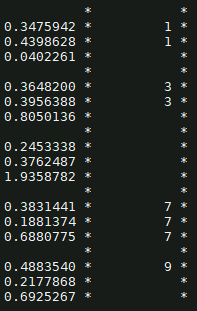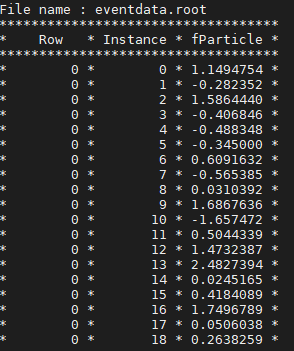# Loop over leaf and draw non-blank entries of another

Hi,

I have a tree with one branch and two leaves x and y. Leaf y has less entries than leaf x. When I scan I have something like this:Left: x, right: y

I would like to draw leaf x only when there is a corresponding value (entry) in leaf y.

For example: entries 1 and 2 (0.34 and 0.43) are examples of entries I want in my histogram, while entry 3 (0.040) I would like to avoid.

Hi @Karl007,

Is your current code using plain `TTree`? I think the easiest way to solve your problem is using an `RDataFrame` filter.
RDataFrame documentation can be accessed here. Also, you can take a look at the tutorials.

IIUC, what you are trying to achieve should be on the line of:

``````// ROOT::EnableImplicitMT();

RDataFrame df("treeName", "file.root");
auto hist = df.Filter([](double x, double y) { return x && y; },
{"x", "y"})
.Histo1D();
// do whatever with `hist`
``````

Cheers,
J.

Hi Javier,

Can this code be run in C++ root?

Hi @Karl007,

Yes, the code above is standard C++11 (actually, note the use of a lambda expression as an argument to the `Filter()` function).

Cheers,
J.

Ok thanks. I will report back.

Hi @jalopezg,

I am trying a simple example to understand how it works first:

``````  ROOT::EnableImplicitMT(); // enable multi-threading
ROOT::RDataFrame df("treeName", "file.root"); // create dataframe

auto hist = df.Filter("leaf.branch > 0").Histo1D("leaf.branch");

hist->Draw();
``````

But it is not working. Is the ‘leaf’ name correct like that? or does x stand for something else?

Hi @Karl007,

If you use the `Filter()` overload that accepts a string (as above), you can directly use a column/branch name there, e.g. `df.Filter("x > 0")`, will yield all the entries whose column `x` is greater than `0`.

Cheers,
J.

Hi @jalopezg,

So I am using the following:

``````	ROOT::RDataFrame df("EventTree", "http://root.cern/files/introtutorials/eventdata.root");
auto h1 = df.Filter("fParticles.fPosX < 5").Histo1D("fParticles.fMomentum");
// get momentum when posX < 5

h1->DrawCopy();
``````

But I am getting an error which I don’t really understand:

``````error: static_assert failed "filter expression returns a type that is not convertible
to bool"
static_assert(std::is_convertible<FilterRet_t, bool>::value,

``````

Do you happen to know the reason for that?

Hi @Karl007,

The assertion is there to ensure that a filter function returns a `bool`, i.e. whether or not an entry is selected.

That said, your filter expression looks right to me, given the schema of the tree contained in `eventdata.root`. Maybe @eguiraud can shed some light here?

Cheers,
J.

Hi,
`fPosX` is an array, so `fPosX < 5` returns an array (which contains the result of the `< 5` comparison for each element). As per the error message, `Filter` expects an expression that returns a boolean in order to decide whether to accept/discard each event.

If you want to draw `fMomentum` for the particles with `fPosX < 5`, you can do it this way:

``````auto h1 = df.Define("good_mom", "fParticles.fMomentum[fParticles.fPosx < 5]").Histo1D("good_mom");
``````

Cheers,
Enrico

Hi @eguiraud,

This is basically the same as what I was trying to do. I think there should be some for loop to check all entries inside indices, because when I scan this is how it looks like:which suggests that I should access indices (starting by zero) then access the entries inside those indices, as you can see the entries for the first index are 0,1,2…18. These are what I am interested in.

What that means is that for every event (i.e. a row in the dataset) you have an array of particles (e.g. 18 particles in the first event). With RDataFrame you specify what you want to do with the array of particle for each event.

For example, my code above selects, for each event, the momentum of the particles that have posX < 5, stores that in a new array called `good_mom`, then draws a histogram of all `good_mom` values for all events.

In your first post you mention that you have, for each event, arrays of different length: le.g. for one event we can see from your first screenshot that leaf y has 2 elements (`{1, 1}`) and leaf x has 3: `{0.3475942, 0.4398628, 0.0402261}`. You say you want to draw x only when there is a corresponding value in leaf y. Then you need to select the first `y.size()` elements from `x` and draw those. Assuming `y.size()` is always smaller or equal than `x.size()`, you can do e.g.:

``````df.Define("my_x", "Take(x, y.size())").Histo1D("my_x")
``````

I hope this clarifies things.
Cheers,
Enrico

This looks like what I am looking for. It works fine with the eventData tree, but when I use it on x and y (where there are missing rows in y), it shows the following error:

``````Error in <TRint::HandleTermInput()>: std::runtime_error caught: Try to take 4 elements but vector has only size 3.
``````

Any ideas?

As per the error message, it appears that sometimes `y.size() > x.size()`. As I mentioned above, my code does not protect against this case.

One way to fix it is to instead use `Take(x, std::min(x.size(), y.size()))`.

Cheers,
Enrico

P.S.

1 Like

Hi Enrico,

it appears that sometimes `y.size() > x.size()`

I could swear that that is impossiblebut anyways it seems to work fine actually.

One last question if I may: just to check the entries I am using:

``````	Double_t filter = df.Define("my_x", "Take(x, y.size())");
cout << "filtered events: " << filter << endl;

``````

But it is showing an error. Is there maybe a different way to see the filtered entries?

You might want to double-check your datayou can actually ask RDF to show the entries in which that happens.

Yes, dataframe objects are not printable. Maybe you want `filter.Display()->Print()` or `std::cout << filter.Display()->AsString() << std::endl;`. See the RDF docs: ROOT: ROOT::RDataFrame Class Reference

Thank you!

Hi @eguiraud,

I am reporting back about using this method:

Take(x, std::min(x.size(), y.size()))

It works fine, but it ignores entries where y.size() > x.size(). In that context I actually lose some events. Is there maybe a different way?

Thanks!

EDIT: also tried:

``````auto c = df.Define(y-variable, y);|

c.Foreach([](ROOT::VecOps::RVec<double_t> &y-variable){ if (y-variable.size()) std::cout << y-variable<< std::endl;}, {x});|

``````

But it prints all of x, and what I want is x when y is true (has value).

What `Take(x, std::min(x.size(), y.size()))` does is to retrieve the first N elements of `x`, where `N = min(x.size(), y.size())`. No events are ignored, and elements of `x` are ignored for which there is no corresponding `y` value. I thought that’s what you needed, I probably misunderstood.

So given `x = {1,2,3}` and `y = {9,42}` what elements of `x` would you like to retrieve?

Cheers,
Enrico

Hi @eguiraud,

Yes, you are right. Sorry for the confusion. I now get it.

To answer your question, I believe that should result in x = {1,2} and it should drop 3.